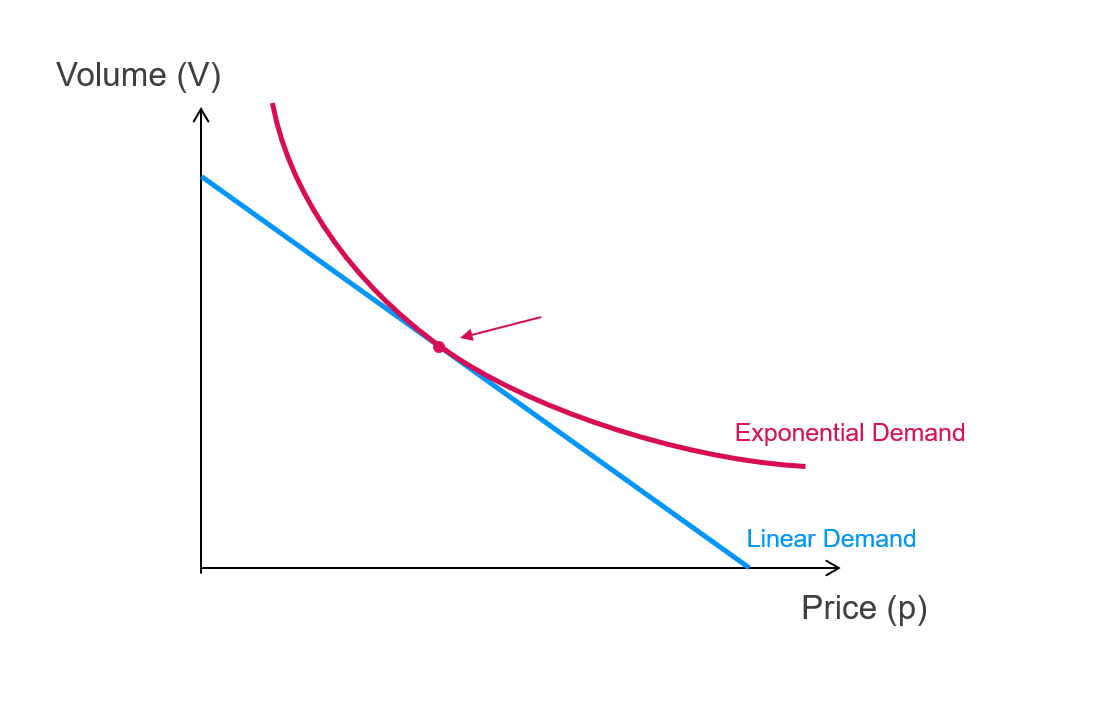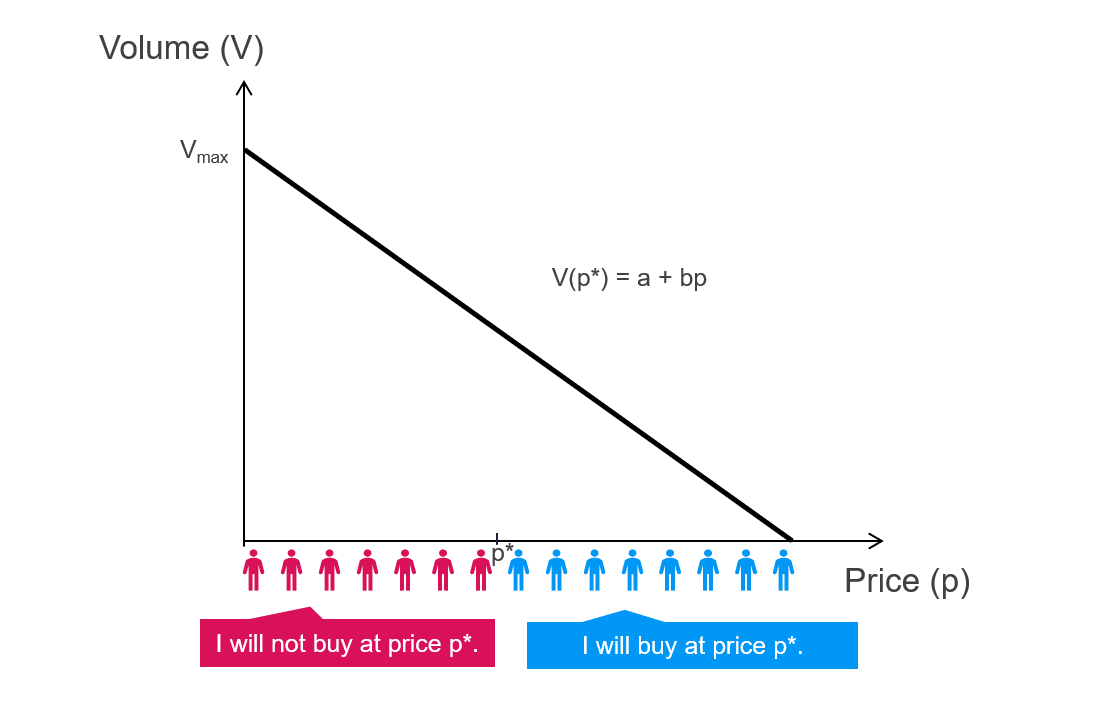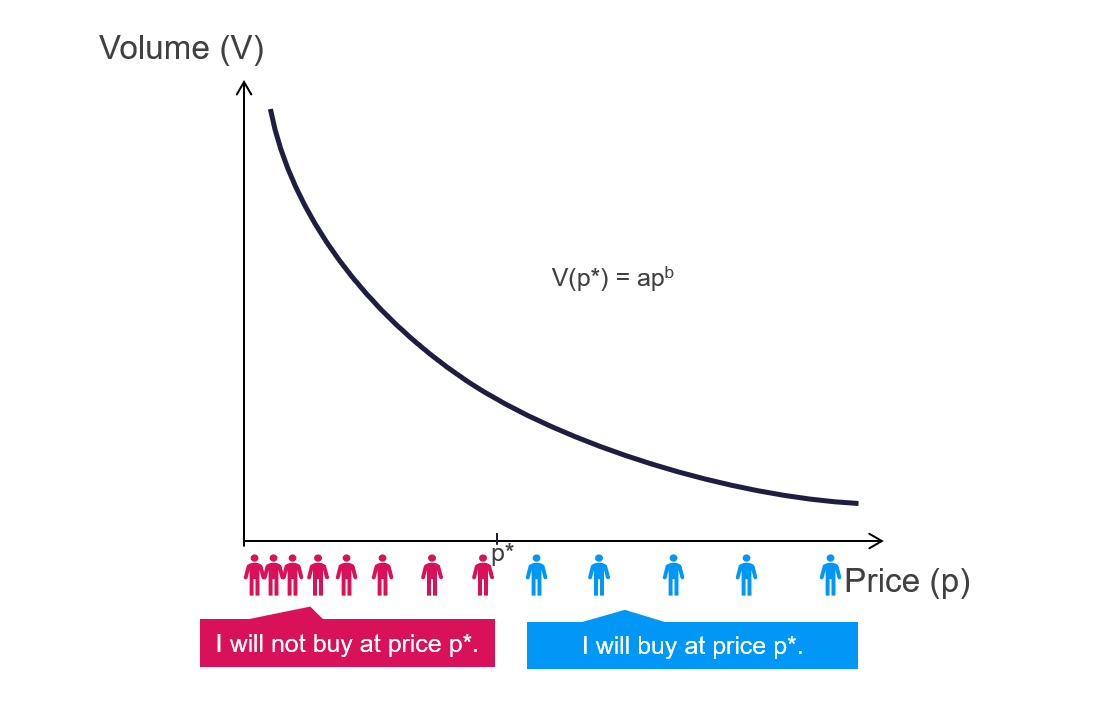First, we want to thank you for all your considerate and thoughtful answers via comments and private messages to help Tim improve his pricing. We have incorporated some of your ideas into our take on the challenge that we want to present in this blog.

We posted this pricing challenge to highlight an inherent difficulty in working with price elasticities to optimize prices. That is the assumption, that the price elasticity contains all relevant information a pricing manager needs to know about how demand reacts to a price change. This assumption is implicit in all elasticity-based price solutions.

To highlight why this is problematic, consider the two most widely used demand functions: linear and exponential demand. Under linear demand, absolute volume changes are proportional to absolute price changes. For example, for each €5 of price increase, you lose 10 units of sales. Under exponential demand, relative volume changes per relative price change remain constant. For example, you lose 10% in volume for every 5% price increase.Figure 1

Figure 1 shows these two demand functions. Pricing on elasticity only works if differences between demand functions and their implications for volume changes are also small and can be neglected — at least for smaller price changes. If the choice of demand function has real implications, then pricing on elasticity can become dangerously misleading. So, let’s investigate how to react to a cost increase as in Tim’s case under linear and exponential demand.

#### Linear demand

Under linear demand, the relation between volume (V) and price (p) is:

where a is the maximum volume, if the product is ‘sold’ at price 0, and b is the slope of the demand function. If sales drop with increasing price, then b is negative. This is the normal case. Figure 2 shows the linear demand function and its underlying assumption about customers’ willingness-to-pay: If customers were lined up, with each customer “standing” on his willingness-to-pay, the distance between customers would remain the same. In other words: You lose the same number of customers if you increase from €10 to €20 or from €70 to €80. Then, price elasticity (ε) is:

and the price optimum is at:

where c is cost.Figure 2

How should Tim react to the cost increase of €5 from €50 to €55 in the Christmas tree example so that he is again in the profit optimum? Following equation (1), half of the absolute cost increase should be passed on as a price increase. That means, that the price of the tree needs to be increased by €2.5 to €102.5. As a result, Tim loses 5% of his sales volume, 3% of revenue, and 10% of profit. This is the best he can do under linear demand.

#### Exponential demand

Under exponential demand, volume (V) is defined as a function of price (p):

where a is a constant, and b is the price elasticity. If sales volumes decrease with price increases, then b is negative. This is the normal case. Figure 3 shows the exponential demand function and its underlying assumption about customers’ willingness-to-pay is: As prices decrease, the distribution of customers densifies constantly. The price optimum is then at:

where c is cost.Figure 3

How should Tim react to the cost increase in the Christmas tree example so that he is again in the profit optimum? Following equation (2), the price needs to be changed by the percentage cost change. That means that if the cost of a Christmas tree increases by €5, that is 10%, then the price of the tree also needs to be increased by 10%, that is by €10 to €110. As a result, Tim loses 17% of his sales volume, 9% of revenue, and 9% of profit. this is the best he can do under exponential demand.

#### Conclusion

It is important to note, that with elastic demand Tim will always lose money if he was in the optimum before the cost increase. However, his price change differs sharply depending on the assumed demand function. Under linear demand, he passes on half of the absolute price change, whereas under exponential demand, he passes on twice the absolute price change to keep his margin constant and to stay in the profit optimum. That is quite a meaningful qualitative difference, and in our example, a significant actual difference of €110 – €102.5 = €7.5.

Linear and exponential demand are extreme cases and can be considered reasonable boundaries of what Tim’s actual demand looks like. Imagine a consultant who has correctly measured Tim’s price elasticity of -2 (see here on measuring price elasticity and its challenges), but cannot be sure about the underlying type of demand function. For her, the honest recommendation to Tim is that he should increase his price by something between €2.5 and €10. If Tim is like most vendors, he would probably have gone for a €5 increase on his own.

In summary, if vendors already price at or near to the price optimum, then pure elasticity-based pricing adds very little in scenarios as the Christmas tree case here – and might actually be harmful if it ignores behavioral considerations, as was correctly pointed out in some of the comments to the riddle. In our webinar next Tuesday (December 18, 2018), we will show how our new method, that uses virtual customers to predict demand reactions to price changes, addresses the shortcomings of pricing on elasticity. Please join if you’re interested.

Want to know more?